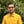Trusted answers to developer questions
Trusted Answers to Developer Questions

Related Tags

java
math
squareroot
communitycreator

# What is Math.sqrt() in Java?Harris Amjad

Grokking Modern System Design Interview for Engineers & Managers

Ace your System Design Interview and take your career to the next level. Learn to handle the design of applications like Netflix, Quora, Facebook, Uber, and many more in a 45-min interview. Learn the RESHADED framework for architecting web-scale applications by determining requirements, constraints, and assumptions before diving into a step-by-step design process.

The sqrt() function returns the square root of a double value sent as a parameter.

Figure 1, below, shows the mathematical representation of the sqrt() function.

Figure 1: Mathematical representation of the sqrt() function

## Syntax

double sqrt(double num)


## Parameter

This function requires a double value as a parameter.

## Return Value

sqrt() returns the square root of a double value sent as a parameter.

If the argument is NaN or 0, then the function returns NaN.

## Example

class JAVA {    public static void main( String args[] ) {        //integer        System.out.println("Math.sqrt(2):");        System.out.println(Math.sqrt(2));        //perfect square root        System.out.println("Math.sqrt(9):");        System.out.println(Math.sqrt(9));        //negative number        System.out.println("Math.sqrt(-4):");        System.out.println(Math.sqrt(-4));    }}

RELATED TAGS

java
math
squareroot
communitycreator

CONTRIBUTORHarris Amjad

Grokking Modern System Design Interview for Engineers & Managers

Ace your System Design Interview and take your career to the next level. Learn to handle the design of applications like Netflix, Quora, Facebook, Uber, and many more in a 45-min interview. Learn the RESHADED framework for architecting web-scale applications by determining requirements, constraints, and assumptions before diving into a step-by-step design process.

Keep Exploring

Learn in-demand tech skills in half the time